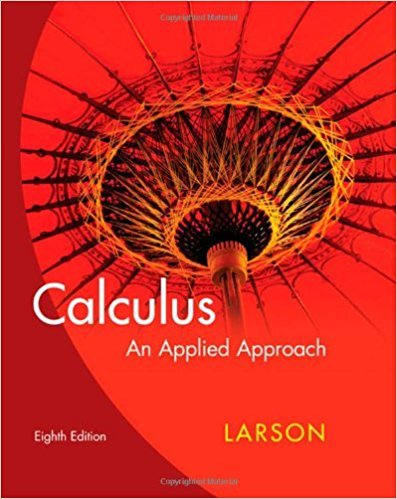×

×

# Solutions for Chapter 1.6: Continuity## Full solutions for Calculus: An Applied Approach | 8th Edition

ISBN: 9780618958252Solutions for Chapter 1.6: Continuity

Solutions for Chapter 1.6
4 5 0 317 Reviews
19
4
##### ISBN: 9780618958252

This textbook survival guide was created for the textbook: Calculus: An Applied Approach , edition: 8. This expansive textbook survival guide covers the following chapters and their solutions. Since 67 problems in chapter 1.6: Continuity have been answered, more than 24064 students have viewed full step-by-step solutions from this chapter. Calculus: An Applied Approach was written by and is associated to the ISBN: 9780618958252. Chapter 1.6: Continuity includes 67 full step-by-step solutions.

Key Calculus Terms and definitions covered in this textbook
• Acceleration due to gravity

g ? 32 ft/sec2 ? 9.8 m/sec

• Coefficient of determination

The number r2 or R2 that measures how well a regression curve fits the data

• Complex fraction

See Compound fraction.

• Components of a vector

See Component form of a vector.

• Distance (in Cartesian space)

The distance d(P, Q) between and P(x, y, z) and Q(x, y, z) or d(P, Q) ((x ) - x 2)2 + (y1 - y2)2 + (z 1 - z 2)2

• Equal complex numbers

Complex numbers whose real parts are equal and whose imaginary parts are equal.

• Explicitly defined sequence

A sequence in which the kth term is given as a function of k.

• Feasible points

Points that satisfy the constraints in a linear programming problem.

• Focal width of a parabola

The length of the chord through the focus and perpendicular to the axis.

• Frequency distribution

See Frequency table.

• Irrational numbers

Real numbers that are not rational, p. 2.

• Logarithmic re-expression of data

Transformation of a data set involving the natural logarithm: exponential regression, natural logarithmic regression, power regression

• Midpoint (on a number line)

For the line segment with endpoints a and b, a + b2

• NINT (ƒ(x), x, a, b)

A calculator approximation to ?ab ƒ(x)dx

• Observational study

A process for gathering data from a subset of a population through current or past observations. This differs from an experiment in that no treatment is imposed.

• Pole

See Polar coordinate system.

• Quotient identities

tan ?= sin ?cos ?and cot ?= cos ? sin ?

• Simple harmonic motion

Motion described by d = a sin wt or d = a cos wt

• Stemplot (or stem-and-leaf plot)

An arrangement of a numerical data set into a specific tabular format.

• Vertices of an ellipse

The points where the ellipse intersects its focal axis.